Think very carefully before answering these questions.

# Algebra Intermediate - Factoring Trinomials [First term is greater than or lesser than 1]

This Math quiz is called 'Algebra Intermediate - Factoring Trinomials [First term is greater than or lesser than 1]' and it has been written by teachers to help you if you are studying the subject at middle school. Playing educational quizzes is a fabulous way to learn if you are in the 6th, 7th or 8th grade - aged 11 to 14.

It costs only \$12.50 per month to play this quiz and over 3,500 others that help you with your school work. You can subscribe on the page at Join Us

Factoring trinomials as you have learned so far, really isn’t hard so long as you pay close attention to first determining whether the problem contains a greatest common factor (GCF) and then working the problem backwards to find the two binomial factors that will give you the trinomial problem. Paying close attention to your mathematical symbols is crucial. However, since we are “factoring” trinomials, we know that multiplication must occur at some point in the solution process.

If you have not already taken the quizzes on Factoring Trinomials [First term is “1”] and Factoring Trinomials [First term is Other than “1”], you really should read those introductions first and take the quizzes as they are lead in quizzes to this quiz. In the first factoring of trinomial quizzes you were give problems where all of the first terms were equal to “1” and the second quiz included numbers greater than the number “1”. In this quiz you will factor trinomials when the first terms may either be greater than the number “1” or lesser than the number “1”. The process is still the same, you just need to be extra careful in watching your mathematical symbols. If you get the symbol wrong, the entire solution will also be wrong.

Since the process is the same here as the past quizzes on factoring trinomials, a practice problem will not be presented. If you do not remember how to work the problem, again, please check the prior quizzes as they will detail this out for you. So with no further delay, check out the next ten trinomial factoring problems and work out the correct solutions.

1.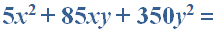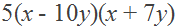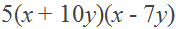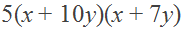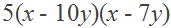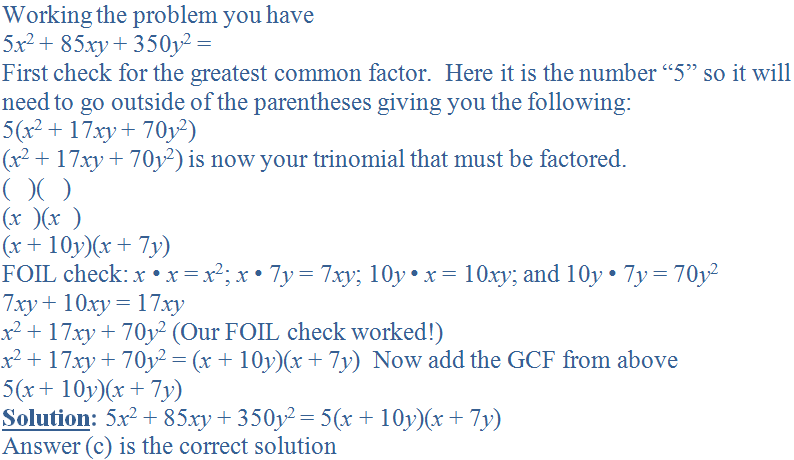2.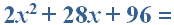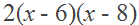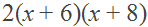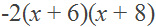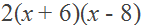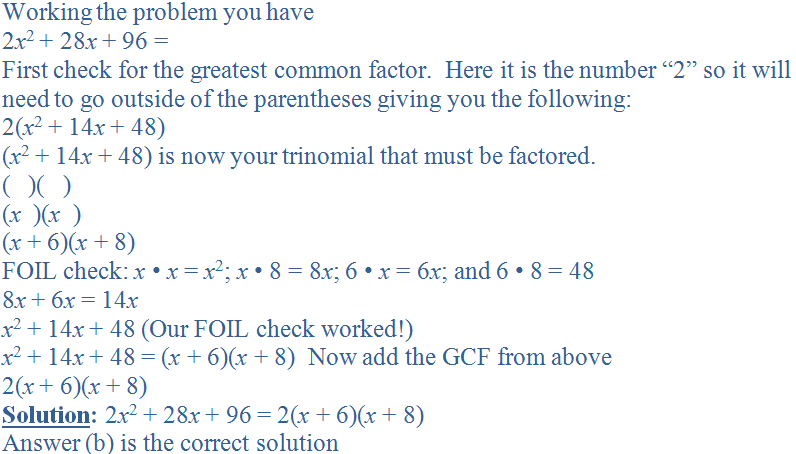3.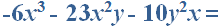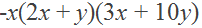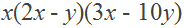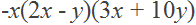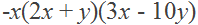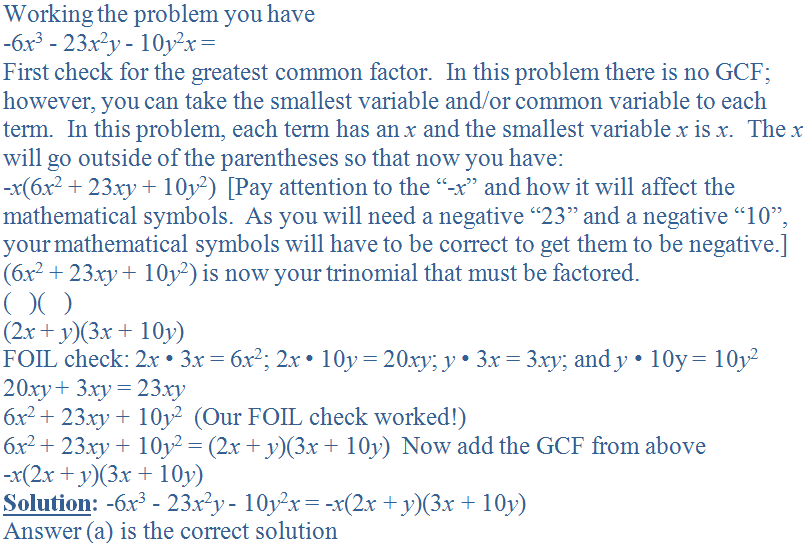4.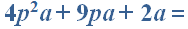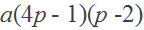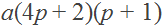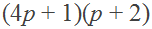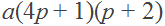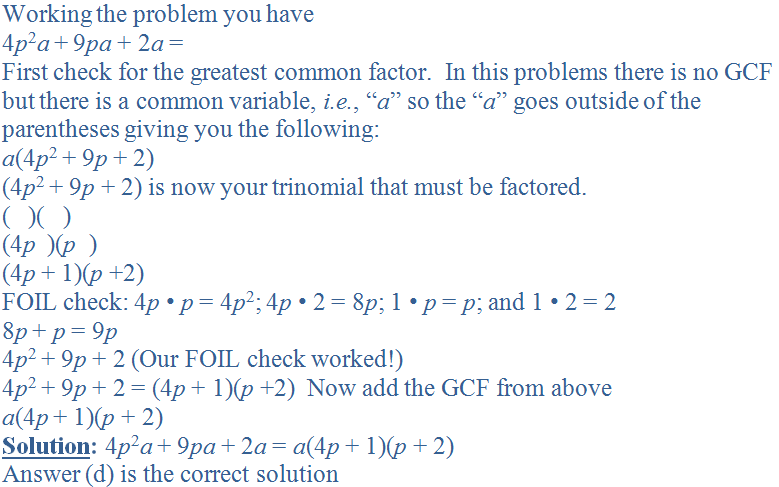5.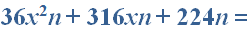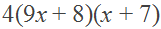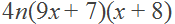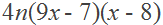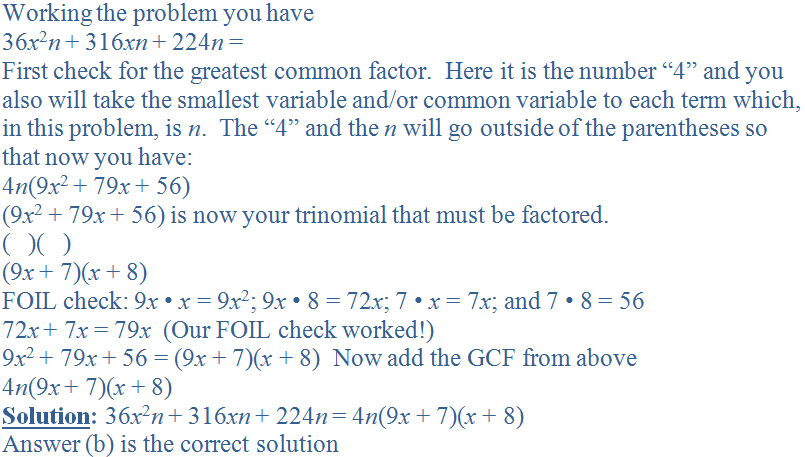6.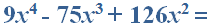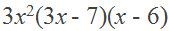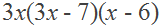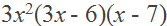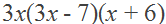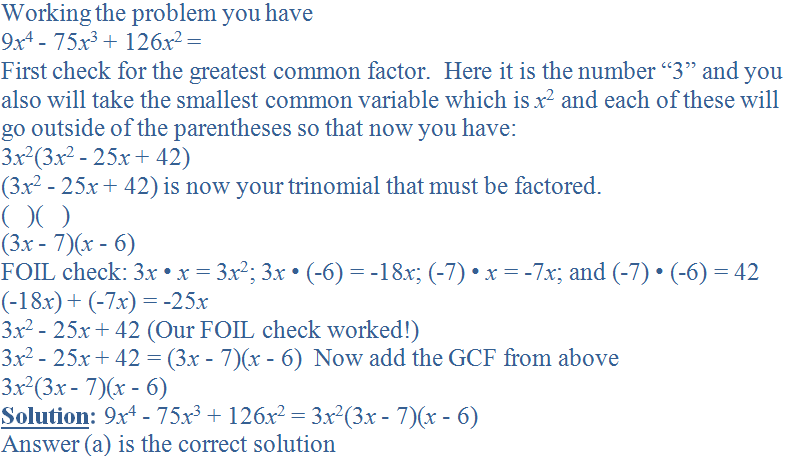7.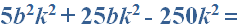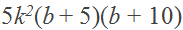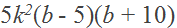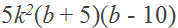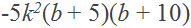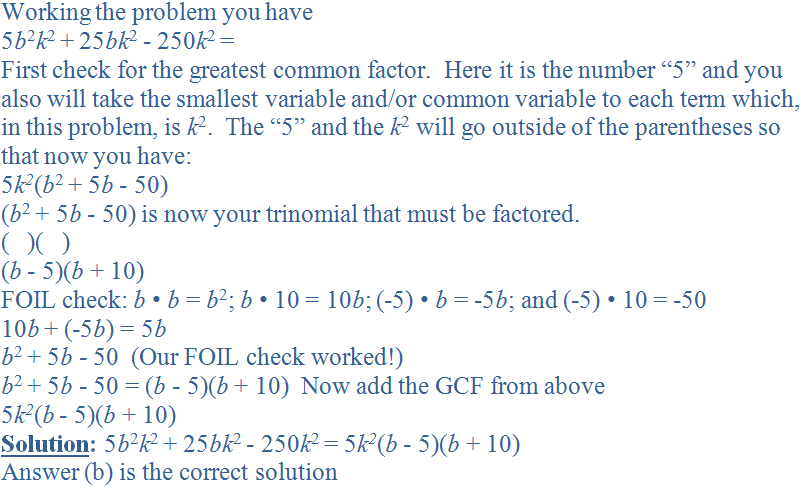8.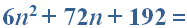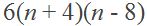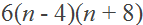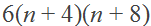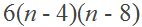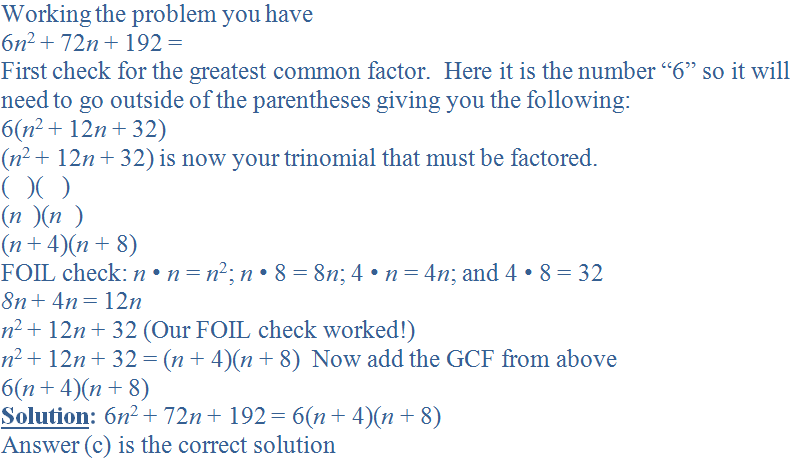9.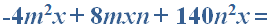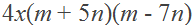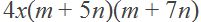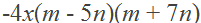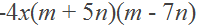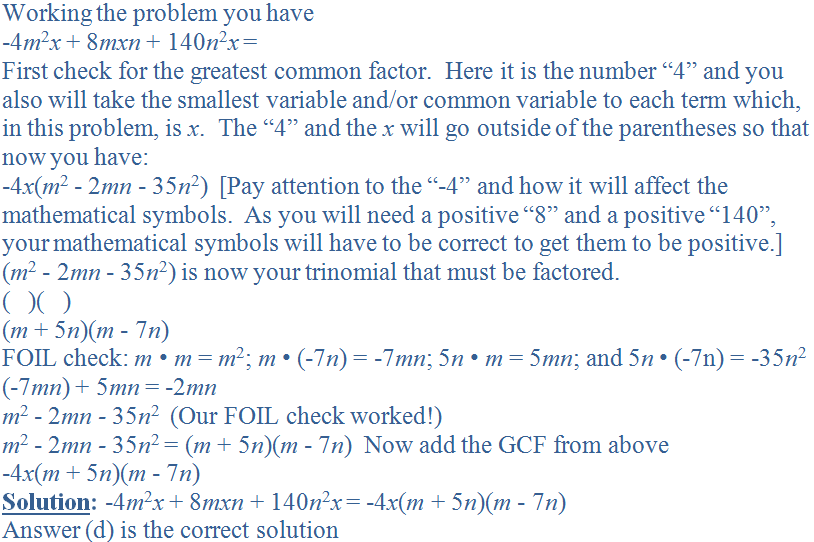10.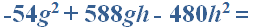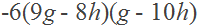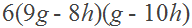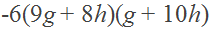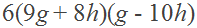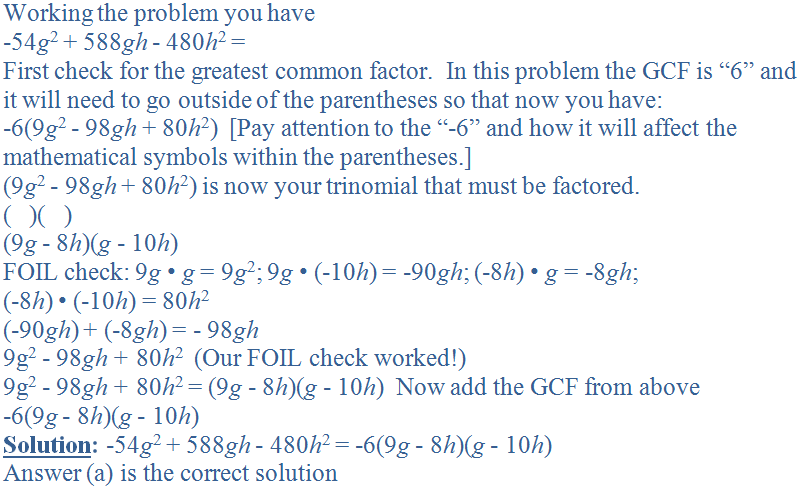Author:  Christine G. Broome